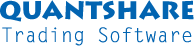#twitter-follow { border: 0;position: fixed; top: 240px; left:0;} #live-support { border: 0;position: fixed; top: 370px; left:0;} #knowledge-base { border: 0;position: fixed; top: 170px; left:0;}

### Artificial Intelligence

Artificial Intelligence

1. Artificial Intelligence

Artificial intelligence is a plug-in that let you build prediction model using neural network and SVM (Support vector machine) systems.

http://en.wikipedia.org/wiki/Neural_network

1.2. Create a prediction model

To create a prediction model, open the 'prediction model' form (Click on 'AI' then 'Prediction'), in the new form, click on 'Add'.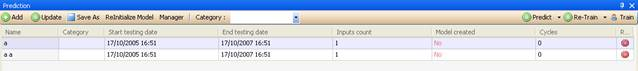1.3. Learning, validation and testing periods

Each prediction model must have three periods.

The first period or the learning period is used to train the model.
The second period or the validation period is used to choose the best model among all the generated models.
The third period or the testing period is used to test the model with new data. (Data on which the model was not trained)
Note that the model is trained only with data from the learning period.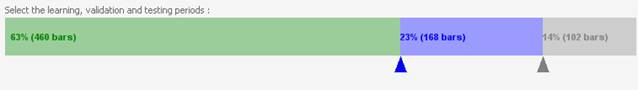To change the learning, validation and testing periods, click on the triangles and move them.

1.4. Learning and validation samples

There are two options:

Normal: The first bars (depending on the learning period) will be associated to the learning samples, and then the next bars will be associated with the validation samples.
Random: The learning and validation periods will be merged and the learning and validation samples will be chosen randomly.

1.5. Periodicity

This option let you choose the period that the prediction item will use.

1.6. Inputs

Inputs are time-series that will be used to train the prediction item.
This is probably the most important step in the creation of the prediction item.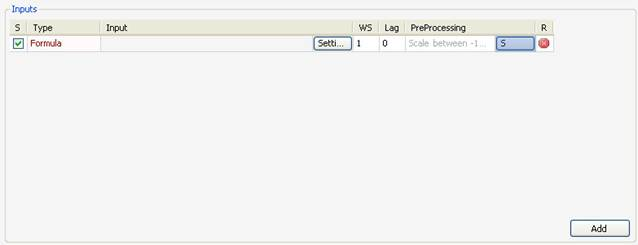Columns:

• Select: The select checkbox indicate whether to enable or disable the current input
• Input Type: Select the input type you would like to use.
• Input: Display information about the input time-series.

·         Settings: Define settings related to the input you have selected

·         WS: Let you create multiple inputs from this input.
Example: if you create 'close' as input and set WS to two, the system will create two time-series. The first one is 'close' and the second one is 'close one bar ago' or 'ref (close, 1)'.

·         Lag: Let you specify the lag to include for the input.
Example: if you create 'close' as input and set Lag to 3, the system will create the following input 'close three bar ago' or 'ref(close, 3)'.

·         Preprocessing: Let you select the pre-processing calculation that will be applied to the input.

·         R: This button is used to remove the selected input.

Input Types:

• Symbol Field: You can choose between open, high, low, close or volume times-series.
• Symbol Return: This creates a formula that returns the performance of one of these time-series: open, high, low, close, volume.
• Formula: This lets you build your own time-series using the formula editor.
• Extern Symbol: This lets you create a time-series based on an external symbol data.
• Database Field: This lets you create a time-series from a field database.

1.7. Output

The output is the time-series that will be predicted.
You can predict a symbol price, a symbol returns or any other time-series.

The output has the same settings as inputs.

The 'WS' column doesn't exists in the output settings.
You can create only one output.

A neural network model has different settings that can dramatically improve or reduce the performance of the prediction model.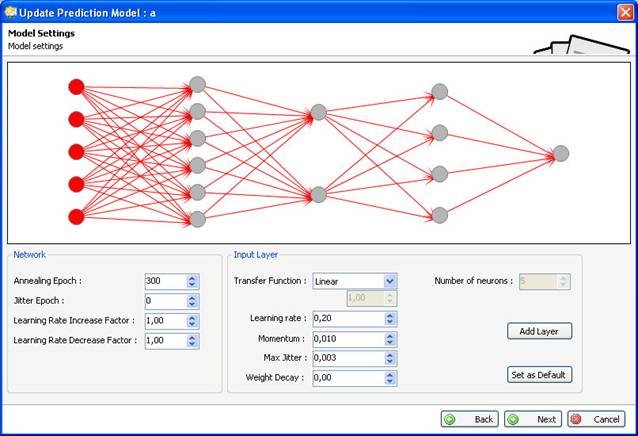1.9. Network

A neural network model is composed of one input layer, one output layer and zero, one or many hidden layers.
Click on the circles to select a layer.

Network settings:

• Annealing Epoch: The epoch at which the learning rate increase or decrease is performed
• Jitter Epoch: The epoch at which the Jitter is performed. Jitter is the process of adding small random noise to weights of all synapses at regular intervals in order to get the network out of a potential local minimum
• Learning Rate Increase Factor: Let the network learn faster in order to improve the global error, value should lies between 1.00 and 1.03
• Learning Rate Decrease Factor: Let the network learn slower in order to improve the global error, value should lies between 0.97 and 1.00

Input layer:
The input layer is the first layer of the neural network model.
The number of inputs determines the number of nodes for this layer.

Hidden layers:
Hidden layers are layers that are always between the input and the output layer.

Output layer:
The output layer is the last layer of the neural network model.
There is only one node in the output layer.
The value that leaves this layer is the predicted value.

Layer settings:

·      Transfer function: Activation or transfer function in a back propagation network defines the way to obtain output of a neuron given the collective input from source synapses.

·      Learning rate: Learning rate is one of the parameters that govern how fast a neural network learns and how effective the training is.

• Momentum: Momentum term associated with each synapse represents its tendency to retain its previous weight change. This factor varies from zero to one.
• Max Jitter: The maximum limit to the random noise added to weights while Jitter, Jitter is the process of adding small random noise to weights of all synapses at regular intervals in order to get the network out of a potential local minimum.
• Weight Decay: Each time the neural network is trained, the weights of synapses are multiplied by this 'Weight Decay'. Weight decay value is usually between 0.9 and 1.

1.10. Filter

The filter lets you create a formula that will be used to reject certain bars from the learning process.
Example: If you want to reject bars where the volume was insignificant then type something like this: 'volume < 100'.

1.11. Symbols

Select the symbols that will be used in the learning process.

1.12. Stop training settings

Specify when to stop the training, there are three options:

• By error value: Stop when the error value reaches a certain level.
• By error change: Stop when the error change goes below a certain value for a determined number of iterations.
• By iterations: Stop after a specific number of iterations.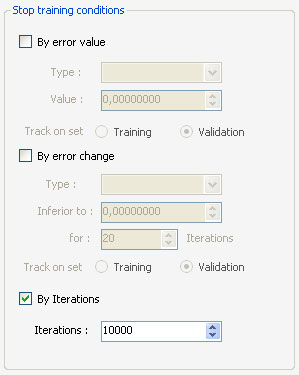1.13. Selecting the best model

Select the model that will be used in prediction among all the models created during the training.

Type: Select the model based on one of these values:

Network Error:

• MSE (Root mean square error)
• POCID (Directional accuracy)
• POCD (Prediction sign accuracy)
• UTHEIL (Coefficient U de Theil)

Track on set: Choose whether to select the best model among the training models or the validation models.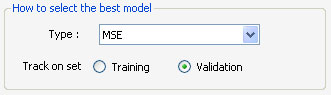1.14. Train a model

In the 'Prediction' form, select an item then click on 'Train', the 'Prediction Progress' form appears.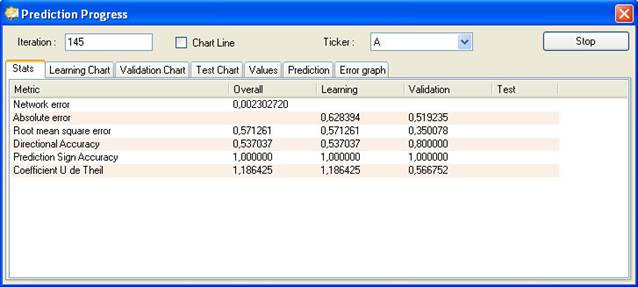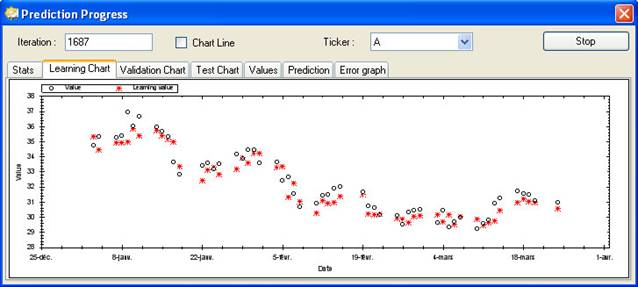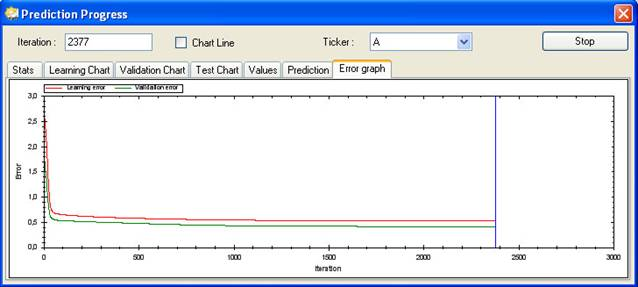The blue line refers to the iteration that produced the best prediction model depending on your settings.

1.15. Prediction

In the 'Prediction' form, select an item then click on the small arrow next to 'Predict'.
You have the choice to choose between 'Predict' and 'Predict a value'.

1.15.1. Predict

This option gives you the ability to predict values for a range of dates.

1.15.2. Predict a value

This option gives you the ability to predict a value for a specific date.

You have the possibility to re-train a prediction model on new data. To do so, click on 'Re-Train' button in the 'Prediction' form.

1.16.1. Re-Training Settings

Re-Training Settings lets you choose the range of dates and the learning and validation samples that will be used for the re-training.

1.17. Update a model

In the 'Prediction' form, select an item then click on 'Update'.
You will lose the data of the prediction model if you update the latter.

1.18. Re-initialize a model

Click on 'Reinitialize Model' in the 'Prediction' form to delete the date of the model.

1.19. Access a model from a formula

Two functions let you access a model data from a formula:

 Predict("category name", "model name") ;

Return a time-series that contains the prediction values.

 PredictSymbol("category name", "model name") ;

Return '1' if the prediction model was trained using the current symbol, otherwise returns '0'.

 Manual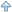Parent   Download Manual in CHM or PDF formatSupport Contact Us Trading Forum How-to Lessons Manual Company About Us Privacy Terms of Use Copyright © 2023 QuantShare.com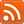Trading financial instruments, including foreign exchange on margin, carries a high level of risk and is not suitable for all investors. The high degree of leverage can work against you as well as for you. Before deciding to invest in financial instruments or foreign exchange you should carefully consider your investment objectives, level of experience, and risk appetite. The possibility exists that you could sustain a loss of some or all of your initial investment and therefore you should not invest money that you cannot afford to lose. You should be aware of all the risks associated with trading and seek advice from an independent financial advisor if you have any doubts.